# Why Topological Data Analysis Can Deal with Complex Data

In our earlier post, we saw that data can exhibit a great deal of complexity, and observed that complexity is often the most significant hurdle to overcome in the analysis process. In this post, we will show how topological data analysis (TDA) can overcome this hurdle, and deal with the great diversity of patterns that occur in real data.

In order to do this, we will first show how TDA deals with data sets which can be handled with traditional techniques.

Linear regression is one of the most frequently used traditional data analytic methods. In the case of two dimensional data, it attempts to approximate a data set by a straight line, as pictured below.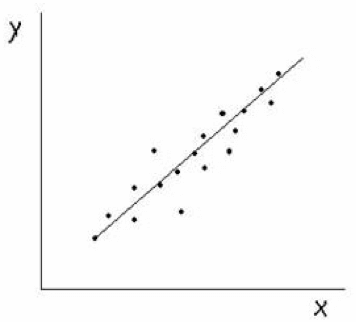In addition to the picture, the line is represented by the equation y = x.

What TDA does is to represent the data set by a network or graph, i.e. a set of nodes (which correspond to sets of data points) and edges between these nodes. In the case of the data set described above, it would produce a network that looks like this.We note that the output to TDA is just a list of nodes and a list of edges connecting those nodes. We obtain a graph on the screen by using a layout algorithm, which will typically produce something that approximates a line, as we see above. Of course, one of the important properties of the xy plot above is the slope of the line, which indicates a particular position of the line in the plane, something that is not recoverable from the TDA graph itself.

However, Ayasdi’s implementation of TDA includes the capability of coloring the graph by variables of interest. For example, if we were to color the graph by the average value of the x-variable, the coloring would look like this.If we colored by the value of the y-variable, it would look like this.The colorings by the x and y variables are identical. This reflects the fact that the line is at a 45 degree angle to the axes, goes through the origin, and most importantly is given by the equation y = x. Suppose instead that the line is not through the origin, and has a different slope. For example, suppose the line looks like this.Its equation is y=x+1. The graph produced by TDA would be the same as the graph above. The coloring by the x-variable would be identical to the colorings above, but the coloring by the y-variable would now look like this.So, the colorings reflect the quantitative properties of the data set. The network reflects the qualitative property that the set is well approximated by a line.

Another very useful method for data analysis is cluster analysis. Suppose we have a data set with two variables, whose scatterplot looks like this.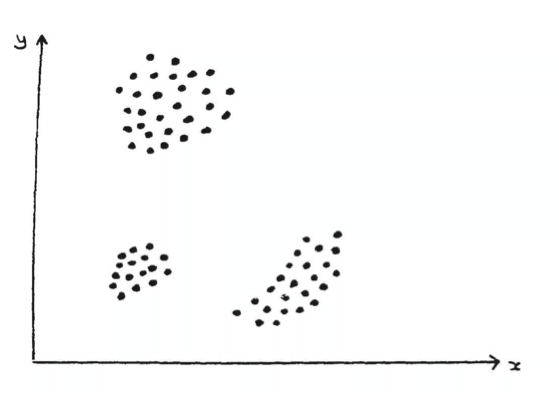Cluster analysis would now recognize that the set breaks up into 3 distinct pieces, and produce three lists of of the data points within each group. TDA would produce a graph that might be laid out like this.The left most graph corresponds to the upper cluster, the middle graph to the lower left cluster, and the rightmost one to the lower right cluster. TDA reflects the cluster decomposition into three pieces, and also retains a bit of information on the shape of the set. As was the case with linear regression above, TDA does not reflect the positioning of the clusters. That can be recovered by coloring the nodes of the graph with the average value of the coordinates in the node. Coloring by the x-variable would look like this.On the other hand, coloring by the y-variable would yield the following image.Again, the coloring can recover the rough positioning of the clusters, and can also indicate a bit of the internal structure within the clusters.

We have looked at how TDA operates on data sets which are successfully addressed by standard methods. Suppose that we are given a data set, again in 2 dimensions, whose scatterplot looks like this.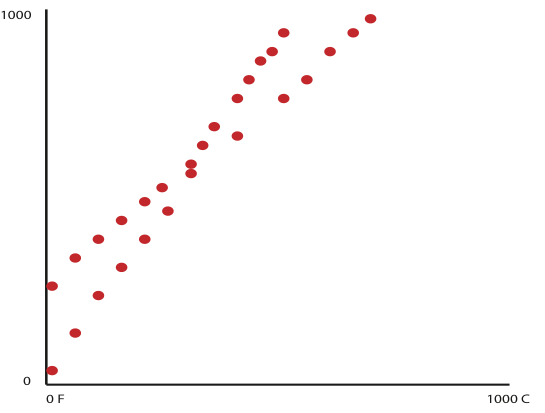This could come from the following situation. We are able to measure temperature on a Fahrenheit scale for a number of locations around the world. In addition, we have a number of other temperature sensors, but have no idea of the nature of the sensors, except that they report back a number reflecting temperature. The x-coordinate in the graph is the Fahrenheit temperature, and the y-coordinate is the measurement reported by the device. If we were to apply linear regression to this data, we would obtain a straight line looking like this.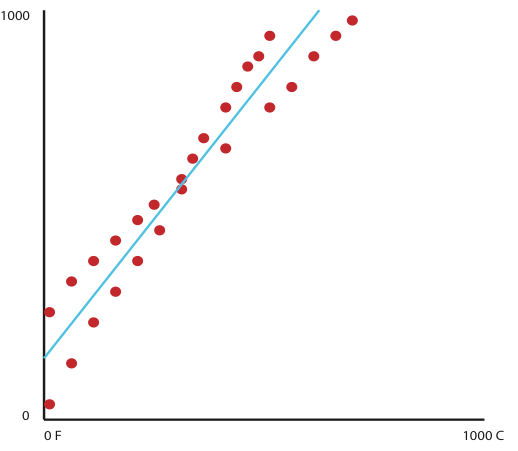The information we could draw from this is that there is a rough correlation between the devices, but that there is substantial scatter around the line, so we could not predict the performance of any of the sensors reliably. Cluster analysis would not yield anything in this case, since the data does not break up into different connected groups in any obvious way. Visual inspection shows in this case that the data appears to lie along two crossing lines. The output of TDA would be a graph that looks like this.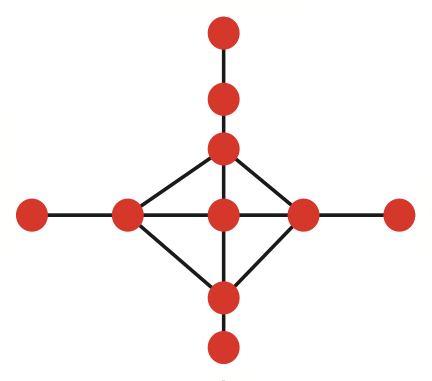Note that it reflects the decomposition into two lines. By now selecting the two distinct segments from the graphs, we can now find that the data breaks naturally into two groups, one consisting of sensors which are measuring temperature in degrees Kelvin and the other measuring in degrees Fahrenheit. Clustering would not discover this decomposition.

As a final example, we will consider one more two–dimensional data set, whose scatterplot looks like this.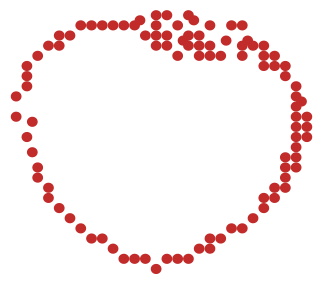We note that the data set is roughly a circle, although it is not perfectly round, and obviously there are some parts where there is an increase in thickness of the “arc”. Linear regression would have difficulty with this situation, since the data does not fit along a line. The regression will tend to produce a horizontal line through the origin, which does not adequately describe the data. Similarly, clustering methods will produce a single cluster.

A TDA representation of this data set might look like this.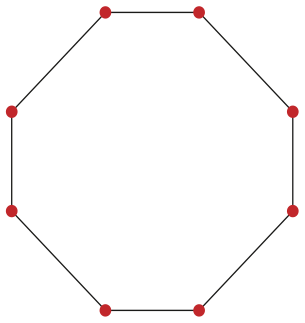Notice that this representation has retained the “loopiness” of the data, while ignoring some of the detail concerning curvature and thickness of the arc. However, one could color the nodes in the network by the x and y coordinates, as above, and obtain a description of the placement of the points in the plane. Alternatively, we might color the nodes of the network by the average value of density, which could capture the thick region of the arc.

The TDA representations of circles and ellipses might be identical, but they can be told apart by the coloring of the nodes by the coordinates.

This is why topological data analysis is so adept at handling complexity. TDA is able to extract information that is “messy” or “tricks” standard approaches.

To be clear, in the example above, a well trained data scientist would find the regression or clustering outputs unsatisfying and would likely dig deeper to attempt to understand the performance more effectively, however, this would be a time consuming process.

TDA on the other hand, can unbundle that complexity easily and therefore both accelerate the insights process as well as deliver a more accurate representation over standard approaches.

To illustrate, let’s go one step further. One frequently used technique is principal component analysis (PCA). PCA is often very useful in producing an interpretable scatterplot based on a dimensionality reduction. However, it sometimes misses interesting information.

Still, complexity, this time in the form of dimensionality can render poor outcomes. Take for example the following:

This image suggests that there are three areas of interest in the data.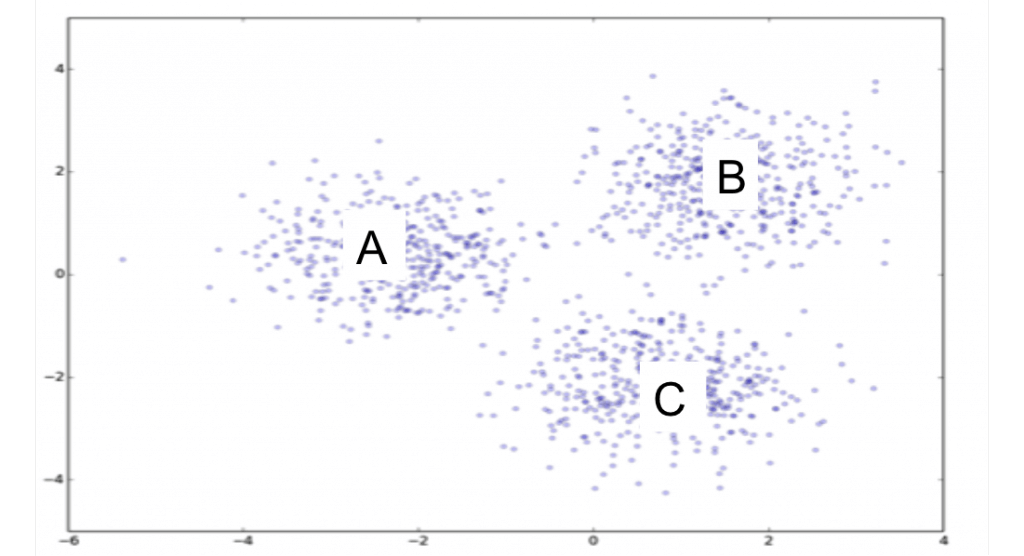What we find with TDA, however, is that there are actually four areas of interest. The reason is that TDA was able to pick up a cluster D that was hidden behind cluster C.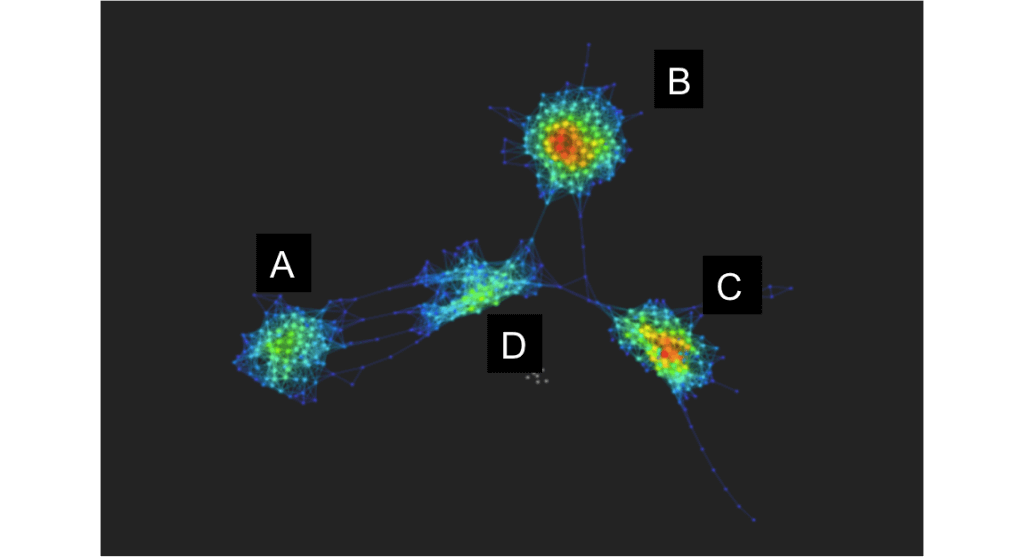The practical implication of mastering complexity is that more of the data’s features are identified. This means that risks are understood, that market regimes are detected, that subtle elements within thousands of surgical events are sourced. With this rapid and comprehensive approach to complexity, the scope of problems that organizations can approach grows commensurately.